# MP Board Class 11th Chemistry Important Questions Chapter 1 Some Basic Concepts of Chemistry

## MP Board Class 11th Chemistry Important Questions Chapter 1 Some Basic Concepts of Chemistry

### Some Basic Concepts of Chemistry Important Questions

Some Basic Concepts of Chemistry Objective Type Questions

Question 1.

Question 1.
Persons dealing with chemistry in Egypt were called:
(a) Scientist
(b) Alchemist
(c) Chemist
(d) Philosphers
(b) Alchemist

Question 2.
Father of Modern chemistry:
(a) Priestly
(c) Kobert Boyle
(d) Rutherford.
(c) Kobert Boyle

Question 3.
The number of molecules present in 16 gm of oxygen gas are:
(a) 6.023 × 1023
(b) 12.044 × 1023
(c) 3.011 × 1023
(d) 1.55 × 1023
(c) 3.011 × 1023Question 4.
The empirical formula of an organic compound is CH2 and its molecular weight is 42. Its molecular formula is:
(a) CH2
(b) C3H6
(C) C2H2
(d) C3H8
(b) C3H6

Question 5.
Modern basis of atomic mass is:
(a) 7N14
(b) 8O16
(c) 6C12
(d) Ar
(c) 6C12

Question 6.
Number of gram atom in 80.25 gm sulphur (at mass of S = 32):
(a) 2.5
(b) 3.2
(c) 5
(d) 10
(a) 2.5

Question 7.
One molal solution contains 1 mole of a solution in:
(a) 1000 gm of the solvent
(b) One litre of the solution
(c) One litre of solvent
(d) 22.4 litre of the solution
(a) 1000 gm of the solventQuestion 8.
Number of atom in 12 gm 6C12:
(a) 6
(b) 12
(c) 6.023 × 1023
(d) 12 × 6.023 × 1023
(c) 6.023 × 1023

Question 9.
Mass of 22.4 litre of nitrogen at N.T.P. will be same as the mass of 22.4 litre of:
(a) Ammonia
(b) Water vapour
(c) Carbon mono – oxide
(d) Hydrogen chloride
(c) Carbon mono – oxide

Question 10.
For the same volume of gases at N.T.P. their:
(a) Number of molecules will be same
(b) Number of atoms will be same
(c) Masses will be same
(d) Densities will be same
(a) Number of molecules will be sameQuestion 11.
On reaction of 2 litre N2 and 2 litre H2 at N.T.P.. The volume of ammonia obtained will be:
(a) 0.665 litre
(b) 1.0 litre
(c) 1.33 litre
(d) 2.66 litre
(c) 1.33 litre

Question 12.
Molarity of pure water:
(a) 18
(b) 50
(c) 55.6
(d) 100
(c) 55.6

Question 13.
At the end of chemical reaction total weight of reactant:
(a) Always increases
(b) Always decreases
(c) Do not change
(d) Always increases or decreases
(c) Do not change

Question 14.
Volume of 0.1 mole gas at N.T.P. will be:
(a) 22.4 litre
(b) 2.24 litre
(c) 0.224 litre
(d) 22,400 litre
(b) 2.24 litre

Question 15.
10 mol of water is:
(a) 1gm
(b) 100 gm
(c) 18 gm
(d) 180 gm
(d) 180 gmQuestion 16.
Atomic number of deuterium is:
(a) 1
(b) 2
(c) 3
(d) 4
(a) 1

Question 17.
Equation: Zn(s) + 2HCli → Zncl2 + H2 ↑ does not provide any information about:
(a) Physical state of reactant and product
(b) Concentration of reactant and product
(c) Thermal change in the reaction
(d) Direction of the reaction
(c) Thermal change in the reaction

Question 18.
Maximum number of molecules are in:
(a) 54 gm nitrogen peroxide
(b) 28 gm carbon mono – oxide
(c) 36 gm water
(d) 46 gm carbon mono – oxide
(a) 54 gm nitrogen peroxide

Question 2.
Fill in the blanks:

1. In ancient time the persons dealing with chemical change were called ………………………
2. Scientist ………………… is known as father of chemistry
3. Actual number of molecules in one grame mole of a gas is ………………………
4. Empirical formula of acetic acid is ……………………
5. …………………. is reciprocal of Avogadro’s number
6. The word chemistry originated from the words ………………….
7. Number of molecules is ………………… in 22.4 litre hydrogen gas at S.T.P.
8. Mass percentage of copper in cupric oxide is ………………………
9. Vapour density of organic compound is 59, where empirical formula is C3H7O. It molecular formula will be …………………….
10. Substance which take part in chemical reaction are called …………………….
11. Indian philosopher ……………………….. postulated that all substance are made up tiny indivisible particles are called ……………………..
12. Atom of different elements having same atomic mass but different atomic number are called ………………………
13. At S.T.P. 5.6 litre of a monoatomic gas contains ……………………………. atoms.
14. Iodized salt contains some amount of ……………………… along with NaCl.

1. Alchemist
2. Berzelius
3. 6.023 × 1023
4. CH2O
5. Avogram
6. Chemia, Chemical reaction
7. 6.023 × 1023
8. 79.8
9. C6H14O2
10. Reactant
12. Isobar
13. 1.5 × 1023
14. Nal.Question 3.

1. Gram molecular mass of O2 is?
2. 16 and 32 gm by weight of oxygen combine seperately with 28 gm N2 to form two oxides N2O and N2O2. This is proved by which law?
3. Number of molecules 6.023 × 1023 present in one gram molecular mass of any substance is called?
4. Number of molecules or atoms presents in one gram molecular mass of a solid matter or gaseous substance is?
5. Atomic mass of sodium is 23 a.m.u. then mass of its 6.023 × 1023 will be?
6. Mass of one mole atoms of an element is equal to its which mass?

1. 32 gram
2. Lawofmultiple proportion
4. 6.023 × 1023
5. 23 gram
6. Gram atomic mass.

Question 4.
Match the following:
(I)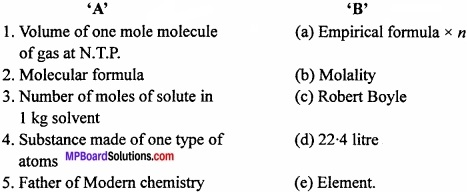1. (d)
2. (a)
3. (b)
4. (e)
5. (c).

(II)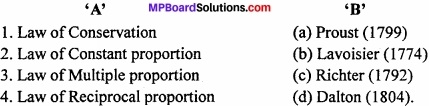1. (b)
2. (a)
3. (d)
4. (c).

Some Basic Concepts of Chemistry Very Short Answer Type Questions

Question 1.
Standard unit of atomic mass is:
Atomic mass unit (a.m.u.).

Question 2.
Empirical formula of benzene is:
CH.

Question 3.
Number of C atom in 1 mole of C02 is:
6.023 × 1023

Question 4.
Significant figure in 0.0500 is:
3.

Question 5.
Molarity of pure water is:
55.6.Question 6.
Volume of 0.1 mole gas at N.T.P. will be:
2.24 L.

Question 7.
Fractional crystallization method used to separate two substance depend on the difference between their:
Solubility.

Question 8.
Formation of 5 oxides of nitrogen (N2O, NO, N2O3, N2O4, N2O5) is according to the law of?
Multiple proportion.

Question 9.
Gram molecular mass of 02 is:
32 gram.

Question 10.
10-15 is expressed for which value?
Femto.

Some Basic Concepts of Chemistry Short Answer Type Questions – I

Question 1.
What is the law of constant proportion?
The law states that, “A chemical compound is always found to be made up of the same elements combined together in the same proportion by weight.” Example: Water from different sources is collected and decomposed. After decomposition in each sample of water, it is found that the weight of hydrogen and oxygen is according to the ratio 2:16 or 1:8.

Question 2.
Write the definitions of gram atomic mass and gram molecular mass?
Gram atomic mass:
Gram atomic mass of an element may be defined as: The mass, which is numerically equal to its atomic mass, expressed in atomic mass units (a.m.u.). It is also called one gram atom of the element. For example, Atomic mass of hydrogen is 1.008 a.m.u. and one gram atom hydrogen is 1.008 gm.
Similarly, Atomic mass of oxygen = 16 a.m.u.
Gram atomic mass = 16 gm.
Gram molecular mass or Gram molecule:
The gram molecular mass of an element is the mass of the substance in gram, which is numerically equal to the molecular mass of substance in atomic mass unit. It is also called one gram molecule of the substance.
For example, molecular mass of carbon dioxide (CO2) = 12 + 2 × 16 = 44 a.m.u.
∴ 1 gram molecule of CO2 = gram molecular mass of CO2 = 44 gm.Question 3.
What is Empirical formula?
Formula which shows the simple ratio of the atoms of various elements present in one molecule of the compound. Importance: Empirical formula gives ratio of elements present in the compound. For example: The ratio of C and H in benzene (C6H6) is 1:1, therefore, its empirical formula is CH.
Other example:

• Empirical formula of acetic acid is = CH2O
• Empirical formula of glucose is = CH2O.

Question 4.
What is mole?
The number of elementary particles present in 12 grams of C – 12 is called mole. It is equal to 6.023 × 1023 particles. This is known as Avogadro number. 6.023 × 1023 atoms, molecules or ions are called one mole atom, one mole molecule or one mole ions respectively.

Question 5.
Define limiting reagent? (NCERT)
The reactant which is completely consumed when a reaction goes to completion is called limiting reagent or limiting reactants because its concentration limits the amount of products formed.

Question 6.
What do you mean by significant figures? (NCERT)
The significant figures in a number are all the certain digits plus one doubtful digit. It depends upon the precision of the scale or instrument used for the measurement.
Example: In 3.03, three digits are significant.

Question 7.
How many significant figures are present in the following: (NCERT)

1. 0.0025
2. 208
3. 5005
4. 126,000
5. 500.0
6. 5

1. 2
2. 3
3. 4
4. 3
5. 4
6. 5.Question 8.
What is rounding off rule? (NCERT)

1. If digit coming after the desired number of significant figures happens to be more than 5, the preceding significant figure is increased by 1.
2. If the digit involved is less than 5, it is neglected as such i.e. the preceding significant figure remains unchanged.

Question 9.
Round off the following up to three significant figures: (NCERT)

1. 34.216
2. 10.4107
3. 0.04597
4. 2808

1. 34.216
2. 10.4107
3. 0.0460
4. 2810.

Question 10.
What is the SI unit of mass, length, time, temperature and amount of substance?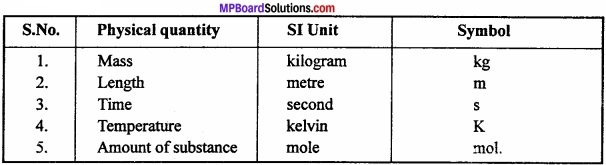Question 11.
Determine the empirical formula an oxide which has 69.9% iron and 30.1 % hydrogen by mass?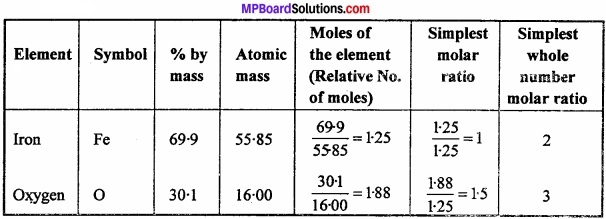Empirical formula = Fe2O3.

Question 12.
Calculate the mass of sodium acetate required to make 500 ml of 0.375 molar aqueous solution. Molar mass of sodium acetate is 80.0245 gm mol-1 (NCERT)
Solution: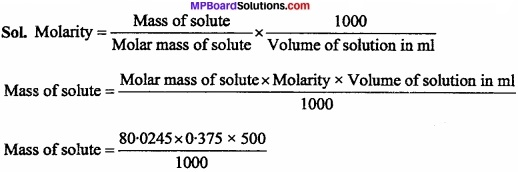Mass of solute = 15.0045 gm.

Question 13.
Calculate the concentration of nitric acid in moles per litre in a sample which has a density 1.41 gm ml-1 and the mass percent of nitric acid in it is being 69%.
Solution:
Molar mass of nitric acid = 63 mol/L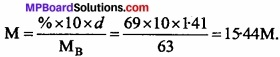Question 14.
How much copper can be obtained from 100 g of copper sulphate (CuSO4)? (Atomic mass of Cu = 63.5 amu). (NCERT)
Solution:
1 mole of CuSO4 contains 1 mole (1 g atom) of Cu
Molar mass of CuSO4 = 63 – 5 + 32 + 4 x 16 = 159.5 g mol-1
Thus, Cu that can be obtained from 159.5 g of CuSO4 = 63.5 g
∴ Cu that can be obtained from 100g of CusO4 = $$\frac { 63.5 }{ 159.5 }$$ × 100 g = 39.81 g.

Question 15.
What is law of multiple proportion? Explain with an example?
Law of multiple proportion: When two elements combines to form two or more compound, a fixed weight of one element reacts with other in the ratio which is simple. Example: CO contains 12 parts by mass of C and 16 parts by mass of oxygen while CO2 contains 12 parts by mass of C and 32 parts by mass of oxygen. Thus, a fixed mass of C(12) combines with oxygen in the ratio 16:32 or 1:2 which is a simple ratio.

Question 16.
What is the law of constant proportion?
The law states that, “A chemical compound is always found to be made up of the same elements combined together in the same proportion by weight.” Example: Water from different sources is collected and decomposed. After decomposition in each sample of water, it is found that the weight of hydrogen and oxygen is according to the ratio 2:16 or 1:8.

Law of constant proportion of matter in light of modern investigations:
Formation of a compound by combination of isotopes of an element with another element puts a question mark on the validity of law of constant proportion. For example, Lead has two isotopes with atomic mass 206 and 208.

First isotope of lead combines with oxygen in the ratio 206:16 while the second reacts in the ratio 208:16. But compounds formed in nature are generally mixture of isotopes and the atomic mass of the element is the average of the atomic masses of various isotopes. Thus, composition of chemical compound remains fixed. Therefore, validity of this law is doubtful.Question 17.
Calculate the amount of carbon dioxide that could be produced when: (NCERT)

1. 1 mole of carbon is burnt in air.
2. 1 mole of carbon is burnt in 16 gm of dioxygen.

The balanced equation for the combustion of carbon in dioxygen/air is –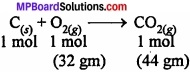1. In air combustion as complete. Therefore, CO2 produced from the combustion of 1 mole of carbon = 44 gm.
2. As only 16 gm of dioxygen is available, it can combine only with 0.5 mole of carbon i.e. dioxygen is the limiting reactant. Hence, CO2 produced = 22 gm.

Question 18.
Determine the molecular formula of an oxide of iron in which the mass percent of iron and oxygen are 69.9 and 30.1 respectively. Given that the molar mass of the oxide is 159.8 gm mol-1. (Atomic mass Fe = 55.85, O = 16.00 a.m.u.) (NCERT)
Solution:
Calculate empirical formula same asEmpirical formula = Fe2O3.
E.F. mass of Fe2O3 = 2 × 55.85 + 3 × 16 = 159.7 gm/mol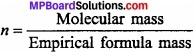= $$\frac{159.8}{159.7}$$ = 1
M.F. = E.F × n
= Fe2O3 × 1
M.F. = Fe2O3.

Some Basic Concepts of Chemistry Short Answer Type Questions – II

Question 1.
Explain the Gay Lussac’s law of gas volume?
Or, One volume of nitrogen combine with 3 volume of hydrogen to give 2 volume of ammonia. Which law supported by this ratio ? Write statement of this law?
Gay Lussac’s Law of Combining Volumes:
When gases react together, they do so in volumes which bear simple ratio to one another and to the volumes of the gaseous products provided all measurements were made under similar condition of temperature and pressure.
For example: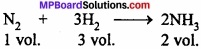Simple ratio of 1:3:2
Hence above example illustrate law of gacous volume.

Question 2.
Define molality of a solution and also write its formula?
Molality of solution may be defined as the number of moles of the solute dis¬solved per 1000 gm of the solvent. It is represented by m.
Molality =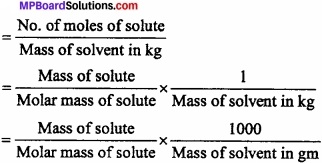Question 3.
Convert the following into SI unit:

1. 28.7 pm
2. 15.15 fts
3. 25365 mg.

Solution:

1. SI uint of length is made28.7 pm × $$\frac { 10^{ -12 } }{ 1pm }$$ = 2.87 × 10-11 m.
2. SI unit of time is second 15.5 µs × $$\frac { 10^{ -6 } }{ 1\mu s }$$ = 1.515 × 10-5s.
3. SI uint of mass is kg 25365 mg × $$\frac { 1g }{ 1000mg }$$ = 2.5365 × 10-2kg.

Question 4.
What is the law of conservation of matter ? What is the present position of this theory?
Law of conservation of matter:
In 1744, Lavoisier gave the law of conservation of matter based on the experiments. This law states that when a chemical reaction takes place, the total mass of the system remains the same before and after the reaction. This law is also referred to as the law of indestructibility of matters, which implies that matter can neither be created nor destroyed by any physical or chemical means.
For example: C + O2 → CO2

Thus 12 grams of carbon combine with 32 gram oxygen to form 44 gram oxygen. In the light of modern investigations, law of conservation of matter: From modem investigations, it is proved that matter can be changed to energy. In some reactions, some energy is produced which causes loss in mass.
According to Einstein’s famous equation of energy – mass relation,
E = mc2

Where, E = Energy (in erg), m = Mass of the substance (in gm), c = Velocity of light (in cm/sec).
In reactions, which we perform in laboratories, very less quantity of energy is absorbed or produced, so the change in mass is very less and is negligible. Such types of changes can be seen clearly in nuclear reaction (atomic bomb). For ordinary reactions, the law is quite valid.Question 5.
Calculate the amount of carbon dioxide that could be produced when:

1. 1 mole of carbon is burnt in air
2. 1 mole of carbon is burnt in 16 g of dioxygen
3. 2 moles of carbon are burnt in 16 g of dioxygen. (NCERT)

The balanced equation for the combustion of carbon in dioxygen/air is:G

1. In air, combustion is complete. Therefore, CO2 produced from the combustion of 1 mole of carbon = 44 g.

2. As only 16 g of dioxygen is available, it can combine only with 0 5 mole of carbon, i.e., dioxygen is the limiting reactant. Hence, CO2 produced = 22 g.

3. Here again, dioxygen is the limiting reactant. 16 g of dioxygen can combine only with 0.5 mole of carbon. CO2 produced again is equal to 22 g.

Question 6.
State the relation between molecular formula and empirical formula?
Or
What is the molecular formula? Explain it with an example?
Molecular formula:
It is defined as the formula which expresses the actual number of atoms of various elements in one molecule of a compound.
Molecular formula = (Empirical formula)n
or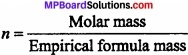Example: The molecular mass of a compound with empirical formula CH2O is 180.= $$\frac{180}{12 + 2 + 16}$$
= $$\frac{180}{30}$$ = 6
Thus, Molecular formula = (Empirical formula)n
= (CH2O)6
= C6H12O6.

Question 7.
Write the difference between Atom and Molecule?
Differences between Atom and Molecule: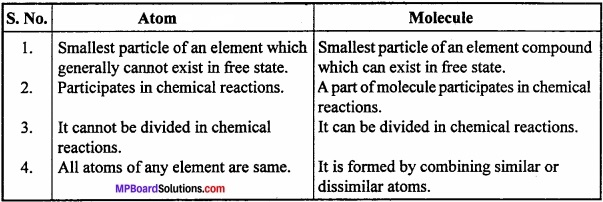Question 8.
Differentiate between molarity and molality?
Differences between Molarity and Molality: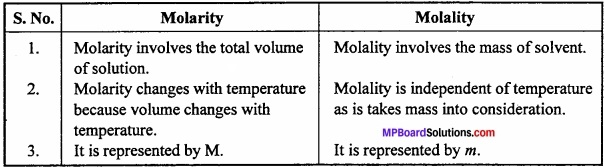Question 9.
What is law of reciprocal proportion? Explain?